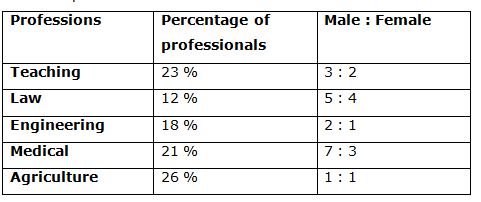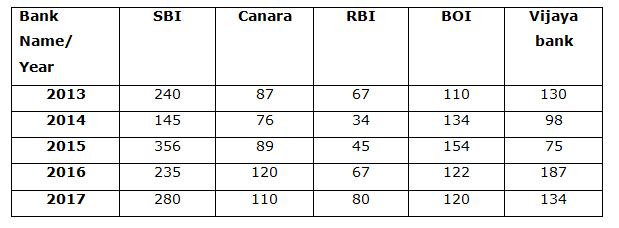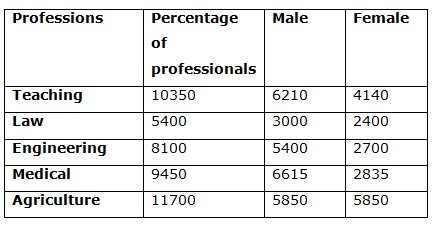# Quantitative Aptitude Questions (Data Interpretation) for NIACL Assistant Mains 2018 Day-208

Dear Readers, NIACL Assistant is conducting Online Examination for the recruitment of Assistant. To enrich your preparation here we have providing new series of Data Interpretation – Quantitative Aptitude Questions. Candidates those who are appearing in NIACL Assistant Mains Exams can practice these Quantitative Aptitude average questions daily and make your preparation effective.

Click “Start Quiz” to attend these Questions and view Solutions

Directions (Q. 1 – 5): Study the following information carefully and answer the given questions:

The following table shows the percentage of total number of professionals in different professions and the ratio of males and females among them.

Total number of professionals = 450001) Find the ratio between the total number of male professions in Teaching and Engineering to that of total number of female professions in Law and agriculture?

a) 285 : 132

b) 563 : 345

c) 387 : 275

d) 413 : 189

e) None of these

2) If the percentage of male and female teaching staffs went for a training is 30 % and 15 % respectively, then find the total number of teaching staffs doesn’t go for any training?

a) 6892

b) 8474

c) 7866

d) 5483

e) None of these

3) Total number of professionals in engineering and medical together is approximately total number of professionals in law and agriculture together?

a) 115 %

b) 103 %

c) 92 %

d) 84 %

e) 127 %

4) Find the average number of female professionals in all the given professions?

a) 3585

b) 4180

c) 5275

d) 2960

e) None of these

5) Find the difference between the total number of male professionals to that of total number of female professionals?

a) 8745

b) 12475

c) 14890

d) 9150

e) None of these

Directions (Q. 6 – 10) Study the following information carefully and answer the given questions:

The following table shows the number of IBPSGUIDE students placed in different banks in different years6) In the year 2013 number of students placed in SBI is what percent of the total number of student placed in Canara bank in the year 2014 and 2015?

a) 150%

b) 145 5/11%

c) 122 3/11%

d) 58 2/3%

e) 165%

7) What is the ratio of average number of students placed in Vijaya bank in the year 2014, 2015 and 2016 to that of students placed in RBI in the year 2013 and 2014?

a) 234:391

b) 47:49

c) 1:2

d) 37:91

e) 240:101

8) If female students placed in SBI in the year 2014 are 25% of students placed in the same bank in the year 2013. Then, what is the ratio of the male to female students placed in SBI in the year 2014?

a) 15:33

b) 31:39

c) 12:17

d) 10:13

e) 21:23

9) What is the difference between the total number of students placed in the year 2013 to the total students placed Vijaya bank in all the given years?

a) 32

b) 28

c) 10

d) 16

e) 24

10) Total number of students placed in RBI and BOI for all the given years together is approximately what percentage more/less than the total number of students placed in SBI and Vijaya bank for all the given years together?

a) 42 % more

b) 70 % less

c) 50 % less

d) 65 % more

e) 36 % less

Direction (1-5) :

Explanation:The total number of male professions in Teaching and Engineering

= > 6210 + 5400 = 11610

The total number of female professions in Law and agriculture

= > 2400 + 5850 = 8250

Required ratio = 11610 : 8250 = 387 : 275

Explanation:

The total number of teaching staffs doesn’t go for any training

= > 6210*(70/100) + 4140*(85/100)

= > 4347 + 3519 = 7866

Explanation:

Total number of professionals in engineering and medical together

= > 8100 + 9450 = 17550

Total number of professionals in law and agriculture together

= > 5400 + 11700 = 17100

Required % = (17550/17100)*100 = 102.63 % = 103 %

Explanation:

The average number of female professionals in all the given professions

= > (4140 + 2400 + 2700 + 2835 + 5850)/5

= > 17925/5 = 3585

Explanation:

The total number of male professionals

= > 6210 + 3000 + 5400 + 6615 + 5850

= > 27075

The total number of female professionals

= > 4140 + 2400 + 2700 + 2835 + 5850

= > 17925

Required difference = 27075 – 17925 = 9150

Direction (6-10) :

Explanation:

Required percentage = [240 / (76+89)]*100

= >145 5/11%

Explanation:

Average students placed in Vijaya bank in the year 2014, 2015 and 2016

= > (98+187+75)/3=360/3 = 120

Average students placed in RBI in the year 2013 and 2014 = 67+34=101

= > 101/2 = 50.5

Required ratio = 120: 50.5 = 1200 : 505 = 240 : 101

Explanation:

Female students in SBI in 2014 =25% of 240 =60

Male students in SBI in 2014 =145-60=85

Required ratio = 60: 85 = 12: 17

Explanation:

Total students placed in the year 2013 = 240+87+67+110+130

= 634

Total students placed Vijaya bank in all the years =130+98+75+187+134

=624

Required difference = 634 – 624 =10

Explanation:

Total students placed in RBI and BOI

= (67+34+45+67+80)+(110+134+154+122+120)

= 293 + 640 = 933

Total students placed in SBI and Vijaya bank

= (240+145+356+235+280) + (130+98+75+187+134)

= 1256 + 624 = 1880

Required % = [(1880 – 933)/1880]*100 = 50.37 % = 50 % less

Daily Practice Test Schedule | Good Luck

 Topic Daily Publishing Time Daily News Papers & Editorials 8.00 AM Current Affairs Quiz 9.00 AM Quantitative Aptitude “20-20” 11.00 AM Vocabulary (Based on The Hindu) 12.00 PM General Awareness “20-20” 1.00 PM English Language “20-20” 2.00 PM Reasoning Puzzles & Seating 4.00 PM Daily Current Affairs Updates 5.00 PM Data Interpretation / Application Sums (Topic Wise) 6.00 PM Reasoning Ability “20-20” 7.00 PM English Language (New Pattern Questions) 8.00 PM